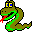#Boost C++ Libraries

...one of the most highly regarded and expertly designed C++ library projects in the world.#Dijkstra Visitor Concept

This concept defines the visitor interface for dijkstra_shortest_paths() and related algorithms. The user can create a class that matches this interface, and then pass objects of the class into dijkstra_shortest_paths() to augment the actions taken during the search.

### Refinement of

Copy Constructible (copying a visitor should be a lightweight operation).

### Notation

 V A type that is a model of Dijkstra Visitor. vis An object of type V. G A type that is a model of Graph. g An object of type G. e An object of type boost::graph_traits::edge_descriptor. s,u,v An object of type boost::graph_traits::vertex_descriptor. DistanceMap A type that is a model of Read/Write Property Map. d An object of type DistanceMap. WeightMap A type that is a model of Readable Property Map. w An object of type DistanceMap.

none

### Valid Expressions

NameExpressionReturn TypeDescription
Initialize Vertex vis.initialize_vertex(u, g) void This is invoked one each vertex of the graph when it is initialized.
Examine Vertex vis.examine_vertex(u, g) void This is invoked on a vertex as it is popped from the queue. This happens immediately before examine_edge() is invoked on each of the out-edges of vertex u.
Examine Edge vis.examine_edge(e, g) void This is invoked on every out-edge of each vertex after it is discovered.
Discover Vertex vis.discover_vertex(u, g) void This is invoked when a vertex is encountered for the first time.
Edge Relaxed vis.edge_relaxed(e, g) void Upon examination, if the following condition holds then the edge is relaxed (its distance is reduced), and this method is invoked.
tie(u,v) = incident(e, g);
D d_u = get(d, u), d_v = get(d, v);
W w_e = get(w, e);
assert(compare(combine(d_u, w_e), d_v));
Edge Not Relaxed vis.edge_not_relaxed(e, g) void Upon examination, if the edge is not relaxed (see above) then this method is invoked.
Finish Vertex vis.finish_vertex(u, g) void This invoked on a vertex after all of its out edges have been added to the search tree and all of the adjacent vertices have been discovered (but before their out-edges have been examined).

### Python

To implement a model of the DijkstraVisitor concept in Python, create a new class that derives from the DijkstraVisitor type of the graph, which will be named GraphType.DijkstraVisitor. The events and syntax are the same as with visitors in C++. Here is an example for the Python bgl.Graph graph type:
```class count_tree_edges_dijkstra_visitor(bgl.Graph.DijkstraVisitor):
def __init__(self, name_map):
bgl.Graph.DijkstraVisitor.__init__(self)
self.name_map = name_map

def edge_relaxed(self, e, g):
(u, v) = (g.source(e), g.target(e))
print "Relaxed edge ",
print self.name_map[u],
print " -> ",
print self.name_map[v]
```

 Copyright © 2000-2001 Jeremy Siek, Indiana University (jsiek@osl.iu.edu) Lie-Quan Lee, Indiana University (llee@cs.indiana.edu) Andrew Lumsdaine, Indiana University (lums@osl.iu.edu)